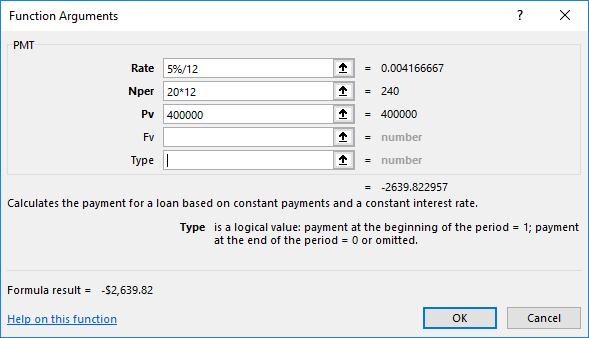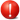# xlf EandA series

## 0. Preliminary

### The EXERCISE - complete the following tasks

Assume that all functions are listed in the Insert Function, User Defined (UDF) category, and are called from the Excel WS.

Required

Number set 1.1 to 1.2

1.1 Area Write a function to calculate the area of a rectangle given the length and width as input arguments. The formula is $$\begin{equation} A = L * W \end{equation}$$ where $A$ is the area, $L$ is the width, and $W$ is the width.

1.2 BSPut Write a function to price a put option. The formula for the price of the put option (Black-Scholes) is $$\begin{equation} P=Xe^{-rT}N(-d_2) - S_0 N(-d_1) \end{equation}$$ where
$$d_1=\frac {log \left( \frac{S_0}{X} \right) + \left( r+ \frac {\sigma^2} {2} \right )T}{\sigma \sqrt{T}}$$ $$d_2=\frac {log \left( \frac{S_0}{X} \right) + \left( r - \frac {\sigma^2} {2} \right )T}{\sigma \sqrt{T}} = d_1 - \sigma \sqrt{T}$$

In equation 2, $S_0$ is the stock price at time 0, $X$ is the exercise price of the option, $r$ is the risk free interest rate, $\sigma$ represents the annual volatility (standard deviation) of the return series for the underlying asset, and $T$ is the time in years, to expiration of the option. Argument names S, X, r, v, and t respectively. The probabilities for $N(\cdot)$ are estimated with the WS NORM.S.DIST function.

To test the function use the values: S = 42, X = 40, r = 0.1, v = 0.2 and t = 0.5. The put price should be 81 cents.

Task 2: Controlling code execution with loops

Number set 2.3 to 2.5. Only 2.5 is included in this version

2.5 xlfNper2 Write a function (named xlfNper2) to calculate the number of repayments (Nper) required to pay off a LOAN of principal (Pv), with interest rate (Rate), and instalment amount (Pmt). Your code must be based on a For...Next loop.

To test the function use the seed values: Pv:= $400,000, Rate:= 5% p.a., Pmt:=$2,639.82 per month (over 20 years). The return values can be derived from this data, ie. ≅240

## 1. VBA functions

### Solution - task 1: Area

Code 1: Function Area
' Write a function to calculate the area of a rectangle given the length and width as input arguments
' Excel WS functions include a function named AREAS (Lookup and Reference category),
' so the name Area is used in this example

'' ==============================================
Function Area(Length As Double, Width As Double) As Double
Area = Length * Width
End Function

'' ==============================================
Sub TestArea()
Dim Ans As Double
Ans = Area(2.4, 12)   ' Returns 28.8
Stop
End Sub



### Solution - task 1: BSPut

Code 2: Function BSPut

' Write a function to price a put option

'' ==============================================
Function BSPut(S As Double, X As Double, r As Double, v As Double, t As Double) As Double

' SYNTAX: BsPut(S,X,r,v,t)
' Arguments:
'       S: Compulsory - Stock price
'       X: Compulsory - eXercise price of the option
'       r: Compulsory - annual rate of interest (risk free)
'       v: Compulsory - annual volatility (standard deviation) of the return series for the underlying asset
'       t: Compulsory - time in years, to expiration of the option

Dim d1 As Double, d2 As Double

With Application.WorksheetFunction
d1 = (Log(S / X) + (r + v ^ 2 / 2) * t) / (v * t ^ 0.5)
d2 = (Log(S / X) + (r - v ^ 2 / 2) * t) / (v * t ^ 0.5)
BSPut = X * Exp(-1 * r * t) * .Norm_S_Dist(-1 * d2, True) _
- S * .Norm_S_Dist(-1 * d1, True)
End With

End Function

'' ==============================================
Sub TestBSPut()
Dim Ans As Double
Ans = BSPut(42, 40, 0.1, 0.2, 0.5) ' Returns 0.808599372900092, rounds up to 0.81
Stop
End Sub



### Solution - task 2: xlfNper2

The seed values: Rate:= 5% p.a., Pmt:= $2,639.82 per month (over 20 years), and Pv:=$400,000, are included in the 1. Loan repayment table of the Repayments worksheet in figure 2. Nper is set to 20 years, and the instalment amount is derived from this data using the WS PMT function (column P and figure 1). PMT(Rate / 12, Nper * 12, PV) returns -$2,639.822957-$2,639.82. The loan is repaid using the rounded amount, thus accumulating as a rounding error in the final instalment.Fig 1: WS PMT function - showing return value and cell value with format (2 decimal places)About the question - the question requires use of a For...Next loop

How to do this can be best illustrated by setting up loan repayment schedule in table form, as displayed by the 2. Loan schedule including running balance in figure 2. The For...Next loop reads each record (in computer memory), keeping count of the number of instalments, until the balance (Pv) is zero.

The suggested solution VBA code is in code 3. See comment line for explanations.

Code 3a: Function xlfNper2
' Write a function (named xlfNper2) to calculate the number of repayments (Nper) required to
' pay off a LOAN of principal (Pv), with interest rate (Rate), and instalment amount (Pmt).
' Include a For...Next loop in your code.

'' ==============================================
Public Function xlfNper2(Rate As Double, _
Pmt As Double, _
Pv As Double) As Long

Const MAXLOOPS As Long = 500        ' max number of instalments
Const ERROR As Double = 2#

Dim AbsPmt As Double
Dim interest As Double
Dim NetReduction As Double          ' reduction in balance
Dim i As Long
Dim n As Long

AbsPmt = Abs(Pmt)
If AbsPmt <= Pv * Rate Then     ' If instalment amount (Pmt) is not sufficient to cover
xlfNper2 = -1               ' current interest then return an error (-1 periods)
Else
n = 0
' Version 3: For...Next loop
For i = 1 To MAXLOOPS
If Pv < 0 Then
Exit For
Else
n = n + 1
interest = Pv * Rate
NetReduction = AbsPmt - interest
Pv = Pv - NetReduction
End If
Next i

'Debug.Print "Print time: " & Time & _
'            ", Closing balance: " & _
'            Format(Pv + AbsPmt, "Currency") & vbNewLine
If Pv + AbsPmt < ERROR Then
xlfNper2 = n - 1
Else
xlfNper2 = n
End If
End If

End Function

'' ==============================================
Sub TestxlfPmt3()
Dim Ans As Double
Ans = xlfNper2(0.05 / 12, -2639.82, 400000)
Stop
End Sub



• This example/solution was developed in Excel 2016 ProPlus 64 bit.